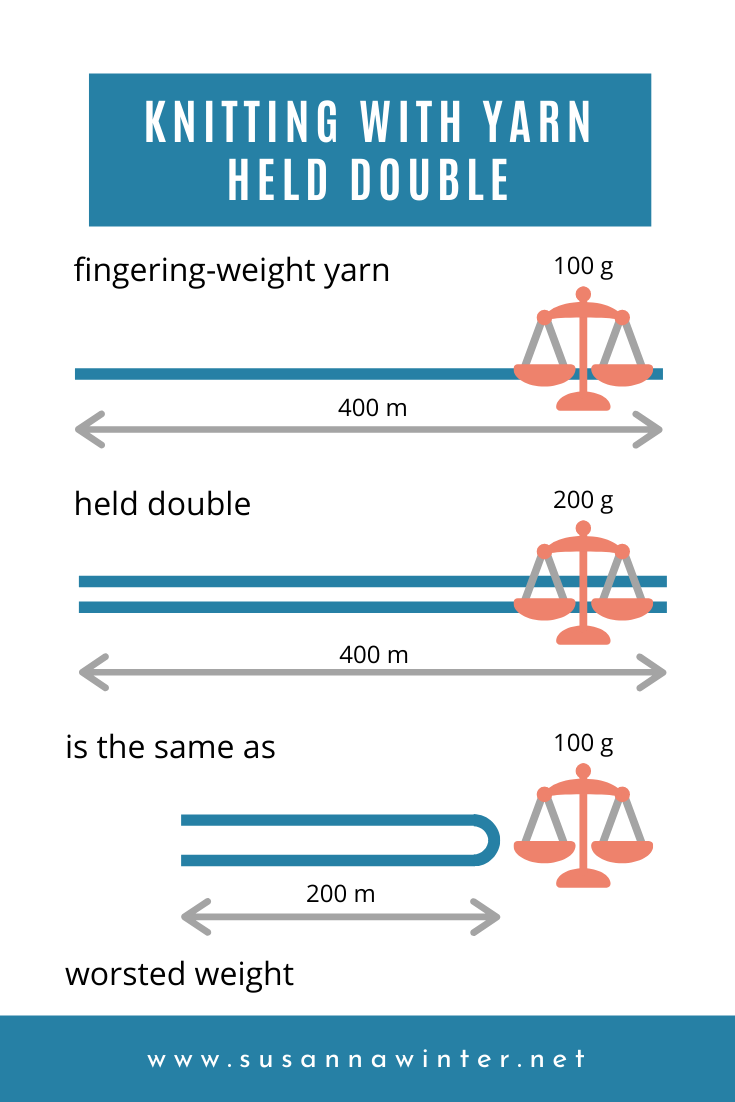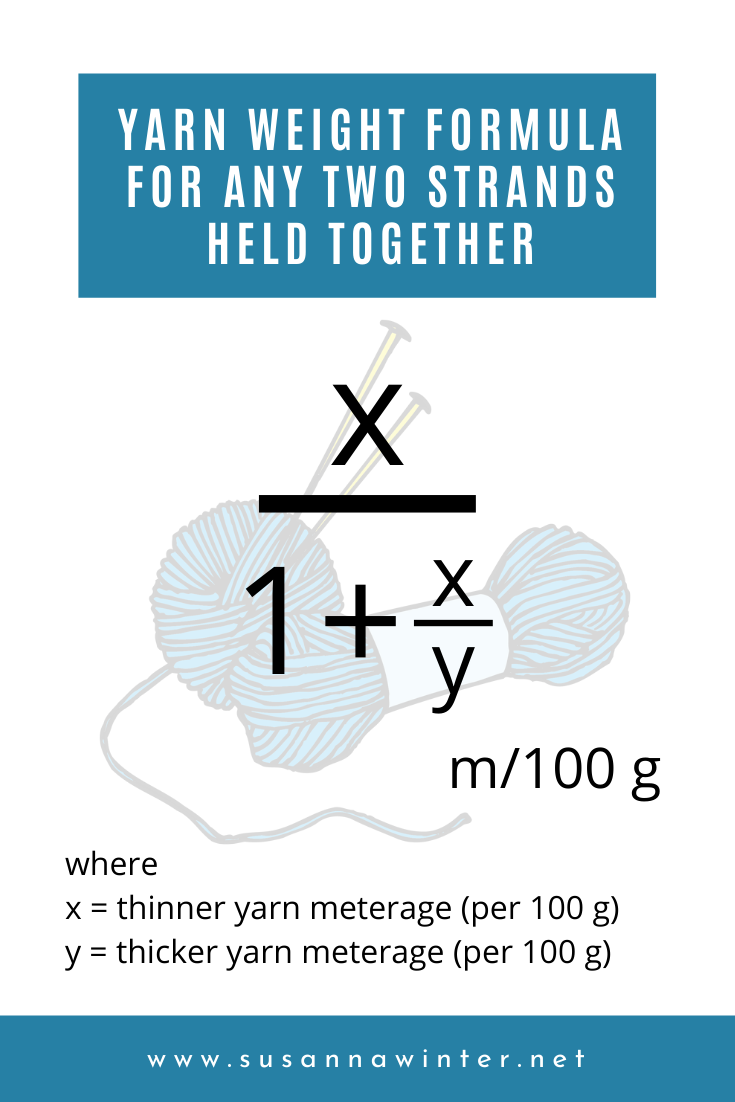top of page## STAY IN THE LOOP

Get tutorials and blog posts delivered right in your email inbox.

# Math for Knitters: Knitting with Yarn Held Double

The recent trend of holding a mohair lace weight together with just about anything has become so popular it's now possible to search patterns on Ravelry specifically for two (or more!) yarns held together. But how do you figure out the yarn weight of two yarns held double? Does it make a difference if they're two different yarns?If you're an experienced yarn addict you can guesstimate the doubled-up yarn weight. If you're not, you can refer to various conversion charts found online, such as this one from mooglyblog.com (which isn't very accurate, by the way).

But there's a better way of doing this that gives you a precise answer every single time, with any combination of yarns, and with any put-up: math.

Side note: I use metric units in my calculations in this post but the math works the same way with imperial.

One Yarn Held Double(d)

Let's start with a simple example: I want to use fingering-weight sock yarn held double — what's the weight of the new yarn?

A very common blend of 75% wool, 25% nylon sock yarn has the meterage of 400 m per a 100 g skein. When held double, the two yarns are essentially stacked on top of each other. The length of the yarn doesn't change — that's still 400 m — but I'm now using double the amount in weight (2 × 100 g = 200 g) to cover the same distance. The two yarns are then 400 m/200 g.Divide both numbers above and below the division bar by 2 and you've got the weight of the doubled-up yarn: 200 m/100 g. You can then look up this number on a yarn weight chart: it's worsted.Yarn weight chart. Sources: ravelry.com, the-knitting-wool-store.com, knittingparadise.com

You can also approach the problem as if you're working from both ends of the same ball: the skein of yarn is doubled over itself so the meterage is halved (400 ÷ 2 = 200 m). The weight of the skein doesn't change; it's still 100 g. Fingering held doubled is therefore the equivalent of 200 m/100 g or worsted-weight yarn.

Rule of Thumb #1: For the same yarn held double, divide meterage (yardage) by 2.

Two Different Yarns Held Together: An Easy Example

But what if I want to use two different weight of yarns held together? Now it gets slightly more complicated.

Let's use the same example yarn, a 400 m/100 g fingering weight, held together with a 800 m/100 g lace weight. First, figure out how many skeins of the thicker (or lower-meterage) yarn it takes to cover the same distance as the thinner (or higher-meterage) yarn. In this case the answer is 800 ÷ 400 = 2 skeins. To go the same distance as the 800 m of lace weight I need to use two skeins, back to back, of the fingering-weight yarn.

Then, calculate the combined weight of the skeins: one skein of lace weight plus two fingering, 100 grams each: (1 + 2) × 100 = 300 g.

Fingering held together with lace weight is then 800 m/300 g. Divide both numbers above and below the division bar by 3 and you get (approximately) 270 m/100 g. That's DK.Different Put-ups of Different Yarns Held Together

That was still pretty straightforward with a made-up example of evenly divisible numbers. Here's a real one: the yarns I used for Milky Oolong are both lace weight but one comes in 50 g skeins, the other in 25 g:

Since the put-ups of the yarns are not the same, first I need to convert the numbers to figure out which of these is the thinner one. Imagining that all yarn comes in 100 g skeins makes these comparisons a lot easier!

• Two skeins of Spinni: 2 × (300 m/50 g) = 600 m/100 g

• Four skeins of Tynn Silk Mohair: 4 × (212 m/25 g) = 848 m/100 g

How many 100-gram skeins of Spinni does it take to cover the same distance as Tynn Silk Mohair? 848 ÷ 600 ≈ 1.41 skeins. The combined weight of the skeins is then (1 + 1.41) × 100 g = 241 g.

The combined yarn weight is 848 m/241 g which (when divided by 2.41) equals approximately to 350 m/100 g. That's sport weight.Cheater Way for Calculating Two Yarns Held Together

Having gone through the trouble of demonstrating the precise way to calculate the weight of two yarns held together, I'm going to teach you a quick-and-dirty cheater way. It's not entirely accurate but it's a lot easier and faster to calculate and gets pretty close to the truth. If you're calculating required yardage for a big project, like a sweater, I recommend using the precise method. But if you're just trying to figure out what two yarns combined would produce, "pretty close" is usually good enough.

First, take the average of the two yarns: add up their different meterages and divide by two. In the above example that would be (600 + 848) ÷ 2 = 724 m

To get the doubled-up meterage, divide again by two: 724 ÷ 2 = 362 m. 362 m/100g is still sport weight. Like I said, pretty close.

Rule of Thumb #2: For two different yarns held together, add up their meterages (yardages) and divide by 4.

The more similar the two yarns are in meterage, the closer the cheater way gets to the precisely calculated result. In fact, if the two yarns are exactly the same, the cheater way produces the same result as Rule of Thumb #1.

The next question is obviously what needle size I should use to knit with the two yarns held together. The short answer (that no one wants to hear) is: knit a gauge swatch.

The slightly longer answer is it depends on my personal gauge, what I intend to knit with the yarns, and the type of fabric I'm going for. A good starting point is to choose a needle size that's 1.5–2 times the recommended needle size listed on the ball band.

Continuing with the example of the 400 m/100 g fingering-weight sock yarn, I usually knit these yarns with 2.5 mm needles. If I were to knit socks with the yarn held double, 1.5 × 2.5 = 3.75 mm needles would produce a firm and dense fabric needed for socks. But if I wanted to knit a sweater instead, 2 × 2.5 = 5 mm needles would give a nice, drapey, and squishy texture. Rule of Thumb #3: To get the needle size for the yarn held double, multiply recommended needle size by 1.5–2.What if the recommended needle sizes are different? Ball bands usually list recommended needle sizes as a range. Take the average of the lower-end numbers and the average of the upper-end ones, then multiply them (separately) by 1.5–2 to get a range for your two-yarns-held-together yarn.

Pin this post!## Related Posts

See All
###### On Instagram

Got a question for me? A great idea for a blog post? Or a knitting tutorial you'd love to see?

Thanks for submitting!